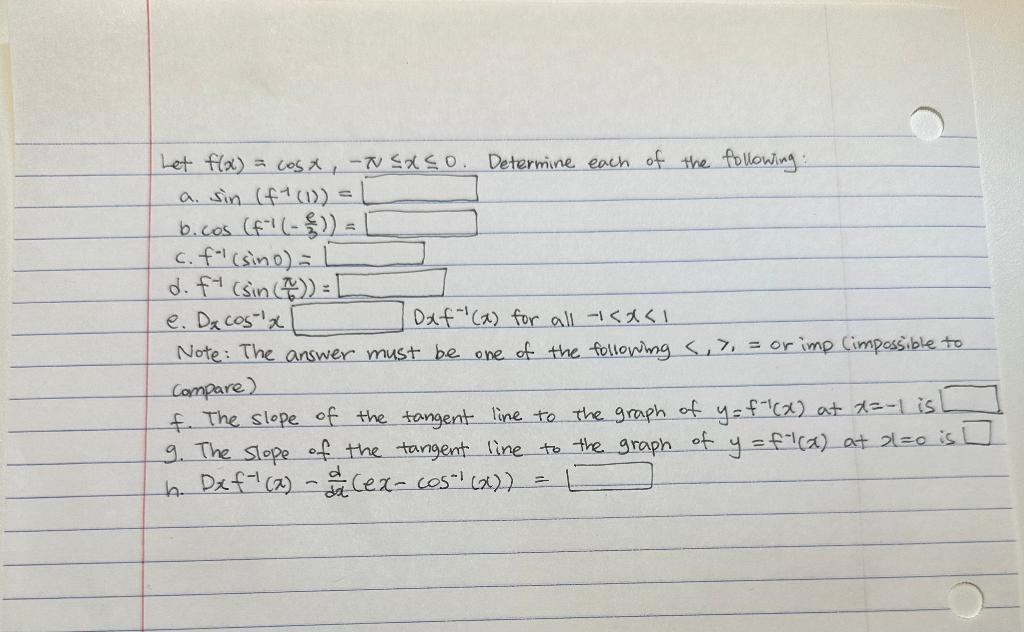Home / Expert Answers / Calculus / calculus-ii-problem-let-f-x-cosx-x0-determine-each-of-the-following-a-sin-f1-pa992

# (Solved): Calculus II Problem Let f(x)=cosx,x0. Determine each of the following: a. sin(f1 ...

Calculus II ProblemLet . Determine each of the following: a. b. c. d. e. for all Note: The answer must be one of the following or imp Cimpossible to compare) f. The slope of the tangent line to the graph of at is 9. The slope of the tangent line to the graph of at is h.

We have an Answer from Expert